Documentation

### This is machine translation

Mouseover text to see original. Click the button below to return to the English version of the page.

## Dealing with Multi-Experiment Data and Merging Models

This example shows how to deal with multiple experiments and merging models when working with System Identification Toolbox™ for estimating and refining models.

### Introduction

The analysis and estimation functions in System Identification Toolbox let you work with multiple batches of data. Essentially, if you have performed multiple experiments and recorded several input-output datasets, you can group them up into a single IDDATA object and use them with any estimation routine.

In some cases, you may want to "split up" your (single) measurement dataset to remove portions where the data quality is not good. For example, portion of data may be unusable due to external disturbance or a sensor failure. In those cases, each good portion of data may be separated out and then combined into a single multi-experiment IDDATA object.

For example, let us look at the dataset iddemo8.mat:

`load iddemo8`

The name of the data object is `dat`, and let us view it.

`dat`
```dat = Time domain data set with 1000 samples. Sample time: 1 seconds Outputs Unit (if specified) y1 Inputs Unit (if specified) u1 ```
`plot(dat)`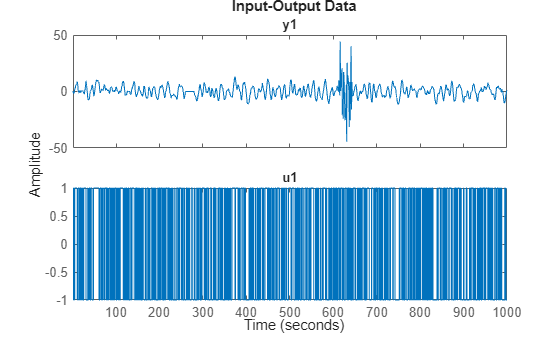We see that there are some problems with the output around sample 250-280 and around samples 600 to 650. These might have been sensor failures.

Therefore split the data into three separate experiments and put then into a multi-experiment data object:

```d1 = dat(1:250); d2 = dat(281:600); d3 = dat(651:1000); d = merge(d1,d2,d3) % merge lets you create multi-exp IDDATA object```
```d = Time domain data set containing 3 experiments. Experiment Samples Sample Time Exp1 250 1 Exp2 320 1 Exp3 350 1 Outputs Unit (if specified) y1 Inputs Unit (if specified) u1 ```

The different experiments can be given other names, for example:

`d.exp = {'Period 1';'Day 2';'Phase 3'}`
```d = Time domain data set containing 3 experiments. Experiment Samples Sample Time Period 1 250 1 Day 2 320 1 Phase 3 350 1 Outputs Unit (if specified) y1 Inputs Unit (if specified) u1 ```

To examine it, use plot, as in `plot(d)`.

### Performing Estimation Using Multi-Experiment Data

As mentioned before, all model estimation routines accept multi-experiment data and take into account that they are recorded at different periods. Let us use the two first experiments for estimation and the third one for validation:

```de = getexp(d,[1,2]); % subselection is done using the command GETEXP dv = getexp(d,'Phase 3'); % using numbers or names. m1 = arx(de,[2 2 1]); m2 = n4sid(de,2); m3 = armax(de,[2 2 2 1]); compare(dv,m1,m2,m3)```The `compare` command also accepts multiple experiments. Use the right click menu to pick the experiment to use, one at a time.

`compare(d,m1,m2,m3)`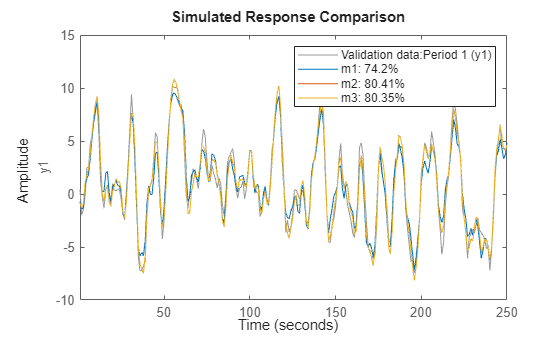Also, `spa`, `etfe`, `resid`, `predict`, `sim` operate in the same way for multi-experiment data, as they do for single experiment data.

### Merging Models After Estimation

There is another way to deal with separate data sets: a model can be computed for each set, and then the models can be merged:

```m4 = armax(getexp(de,1),[2 2 2 1]); m5 = armax(getexp(de,2),[2 2 2 1]); m6 = merge(m4,m5); % m4 and m5 are merged into m6```

This is conceptually the same as computing `m` from the merged set `de`, but it is not numerically the same. Working on `de` assumes that the signal-to-noise ratios are (about) the same in the different experiments, while merging separate models makes independent estimates of the noise levels. If the conditions are about the same for the different experiments, it is more efficient to estimate directly on the multi-experiment data.

We can check the models `m3` and `m6` that are both ARMAX models obtained on the same data in two different ways:

`[m3.a;m6.a]`
```ans = 2×3 1.0000 -1.5034 0.7008 1.0000 -1.5022 0.7000 ```
`[m3.b;m6.b]`
```ans = 2×3 0 1.0023 0.5029 0 1.0035 0.5028 ```
`[m3.c;m6.c]`
```ans = 2×3 1.0000 -0.9744 0.1578 1.0000 -0.9751 0.1584 ```
`compare(dv,m3,m6)`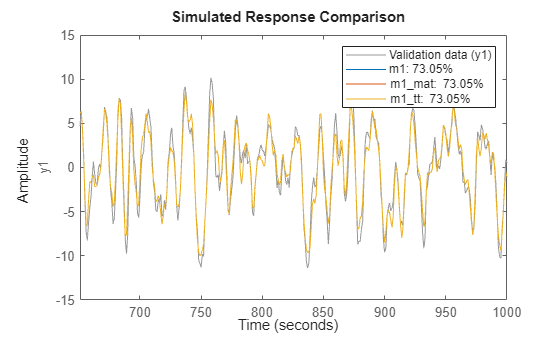### Case Study: Concatenating Vs. Merging Independent Datasets

We now turn to another situation. Let us consider two data sets generated by the system m0. The system is given by:

`m0`
```m0 = Discrete-time identified state-space model: x(t+Ts) = A x(t) + B u(t) + K e(t) y(t) = C x(t) + D u(t) + e(t) A = x1 x2 x3 x1 0.5296 -0.476 0.1238 x2 -0.476 -0.09743 0.1354 x3 0.1238 0.1354 -0.8233 B = u1 u2 x1 -1.146 -0.03763 x2 1.191 0.3273 x3 0 0 C = x1 x2 x3 y1 -0.1867 -0.5883 -0.1364 y2 0.7258 0 0.1139 D = u1 u2 y1 1.067 0 y2 0 0 K = y1 y2 x1 0 0 x2 0 0 x3 0 0 Sample time: 1 seconds Parameterization: STRUCTURED form (some fixed coefficients in A, B, C). Feedthrough: on some input channels Disturbance component: none Number of free coefficients: 23 Use "idssdata", "getpvec", "getcov" for parameters and their uncertainties. Status: Created by direct construction or transformation. Not estimated. ```

The data sets that have been collected are `z1` and `z2`, obtained from m0 with different inputs, noise and initial conditions. These datasets are obtained from iddemo8.mat that was loaded earlier.

`pause off`

First data set:

`plot(z1) %generates a separate plot for each I/O pair if pause is on; showing only the last one here`The second set:

`plot(z2) %generates a separate plot for each I/O pair if pause is on; showing only the last one here`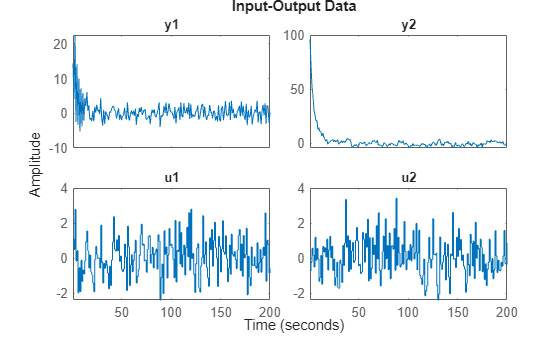If we just concatenate the data we obtained:

`zzl = [z1;z2]`
```zzl = Time domain data set with 400 samples. Sample time: 1 seconds Outputs Unit (if specified) y1 y2 Inputs Unit (if specified) u1 u2 ```
`plot(zzl)`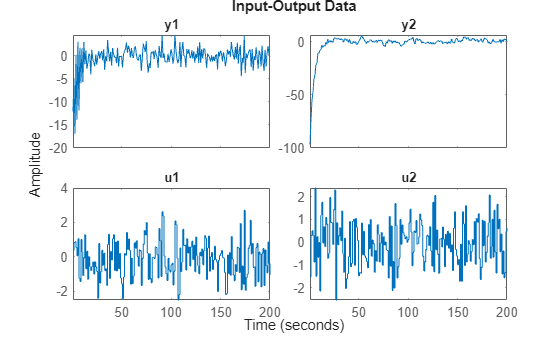`pause on`

A discrete-time state-space model can be obtained by using `ssest`:

`ml = ssest(zzl,3,'Ts',1, 'Feedthrough', [true, false]);`

Compare the bode response for models m0 and ml:

```clf bode(m0,ml) legend('show')```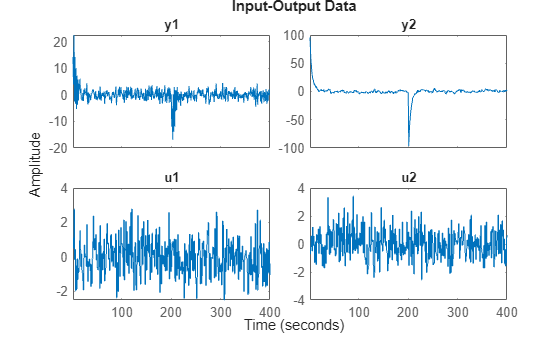This is not a very good model, as observed from the four Bode plots above.

Now, instead treat the two data sets as different experiments:

`zzm = merge(z1,z2)`
```zzm = Time domain data set containing 2 experiments. Experiment Samples Sample Time Exp1 200 1 Exp2 200 1 Outputs Unit (if specified) y1 y2 Inputs Unit (if specified) u1 u2 ```
```% The model for this data can be estimated as before (watching progress this time) mm = ssest(zzm,3,'Ts',1,'Feedthrough',[true, false], ssestOptions('Display', 'on'));```

`Let us compare the Bode plots of the true system (blue)`

`the model from concatenated data (green) and the model from the`

`merged data set (red):`

```clf bode(m0,'b',ml,'g',mm,'r') legend('show')```The merged data give a better model, as observed from the plot above.

### Conclusions

In this example we analyzed how to use multiple data sets together for estimation of one model. This technique is useful when you have multiple datasets from independent experiment runs or when you segment data into multiple sets to remove bad segments. Multiple experiments can be packaged into a single IDDATA object, which is then usable for all estimation and analysis requirements. This technique works for both time and frequency domain iddata.

It is also possible to merge models after estimation. This technique can be used to "average out" independently estimated models. If the noise characteristics on multiple datasets are different, merging models after estimation works better than merging the datasets themselves before estimation.

## Related Topics

##### SupportGet trial now